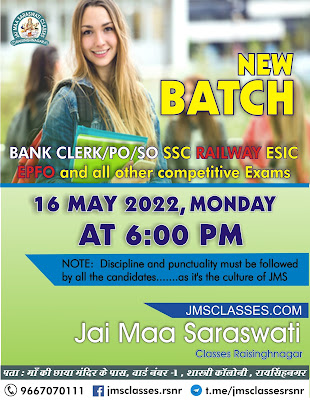### English Quiz : 02-04-2021

Directions (1-10): In the following passage there are blanks, each of which has been numbered. These numbers are printed below the passage and against each, some words are suggested, one of which fits the blank appropriately. Find out the appropriate word in each case.

Q1.
(a)Baseless
(b)Irrational (c)Rational
(d)Scientific
(e)Unreasonable

C

Directions (1-10): In the following passage there are blanks, each of which has been numbered. These numbers are printed below the passage and against each, some words are suggested, one of which fits the blank appropriately. Find out the appropriate word in each case.

Q2.
(a)Artificially
(b)Thoroughly
(c)Recklessly
(d)Negligently
(e)Carelessly

B

Directions (1-10): In the following passage there are blanks, each of which has been numbered. These numbers are printed below the passage and against each, some words are suggested, one of which fits the blank appropriately. Find out the appropriate word in each case.

Q3.
(a)Scatter
(b)Distribute
(c)Twist
(d)Disperse
(e)Accumulate

E

Directions (1-10): In the following passage there are blanks, each of which has been numbered. These numbers are printed below the passage and against each, some words are suggested, one of which fits the blank appropriately. Find out the appropriate word in each case.

Q4.
(a)Integrated
(b)Diverge
(c)Sequential
(d)Constituted
(e)Confused

A

Directions (1-10): In the following passage there are blanks, each of which has been numbered. These numbers are printed below the passage and against each, some words are suggested, one of which fits the blank appropriately. Find out the appropriate word in each case.

Q5.
(a) Caught
(b) Unit
(c) Separated
(d)Fused
(e) Disconnected

D

Directions (1-10): In the following passage there are blanks, each of which has been numbered. These numbers are printed below the passage and against each, some words are suggested, one of which fits the blank appropriately. Find out the appropriate word in each case.

Q6.
(a)Ambiguity
(b)Belief
(c)Illusion
(d)Uncertainty
(e)Queue

B

Directions (1-10): In the following passage there are blanks, each of which has been numbered. These numbers are printed below the passage and against each, some words are suggested, one of which fits the blank appropriately. Find out the appropriate word in each case.

Q7.
(a)Reassurance
(b)Supposition
(c)Evidence
(d)Conception
(e)Intuition

E

Directions (1-10): In the following passage there are blanks, each of which has been numbered. These numbers are printed below the passage and against each, some words are suggested, one of which fits the blank appropriately. Find out the appropriate word in each case.

Q8.
(a)Indefinite
(b)Obvious
(c)Immeasurable
(d)Limited
(e)Awful

D

Directions (1-10): In the following passage there are blanks, each of which has been numbered. These numbers are printed below the passage and against each, some words are suggested, one of which fits the blank appropriately. Find out the appropriate word in each case.

Q9.
(a)Applications
(b)Records
(c)Distractions
(d)Researches
(e)Willingness

C

Directions (1-10): In the following passage there are blanks, each of which has been numbered. These numbers are printed below the passage and against each, some words are suggested, one of which fits the blank appropriately. Find out the appropriate word in each case.

Q10.
(a)Genuine
(b)Inauthentic
(c) Original
(d)Accurate
(e)Unanimous

B

Ⓒ 2019. JMS Classes Raisinghnagar
✆ 9667070111

Designed By : Satnam Gill